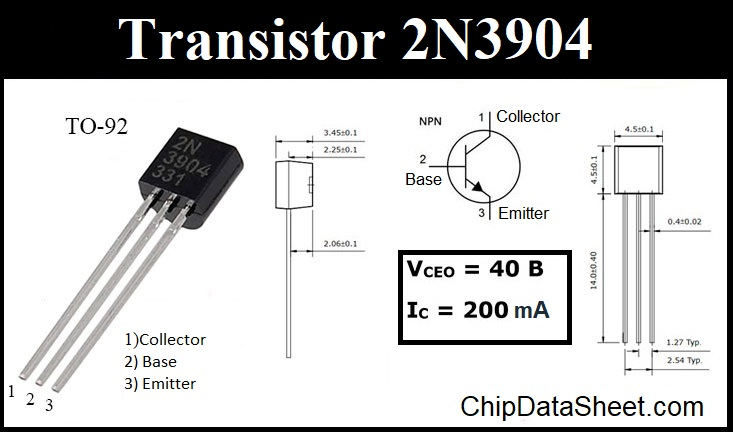# Transistor 2N3904: specifications, pinout and equivalents

Specifications Bipolar junction transistors 2n3904 (BJT) indicate that it is most often used in television and consumer electronics as a light load switch. It can also be used as a simple amplifier or stabilizer, depending on the electronic circuit.

## Pinout 2N3904

The pinout 2N3904 will be considered in the TO-92 Package. There are also equivalents in SOT-223 and SOT-23 (MMBT3904), they are marked as “1A”. If you position the transistor so that the side with the marking is directly in front of you, and the legs look down, then the legs will be located like this:

• the collector is on the left;
• the base is in the middle;
• the emitter is on the right.

The appearance, geometric dimensions and pin diagram of 2N3904 are shown in the figure.## Specifications

Now consider the maximum specs, since they are the most important and they should be looked at when looking for a replacement and designing new devices. The tests were carried out at a temperature of +25°C.

Maximum Specifications 2N3904:

• Max Collector-Base Voltage – 60 V;
• Max Collector-Emitter Voltage |Vce| – 40 V;
• Max Emitter-Base Voltage (VEBO) – 6V;
• Max Collector Current(IC) – 200mA;
• Total power dissipation – 625 Milliwatt (mW);
• The maximum storage and operation temperature must be in the range of -50 … +150°C.

Attention! The table below scrolls.

 Electrical characteristics 2N3904 (T = +25°C) PARAMETER SYMBOL TEST CONDITIONS min max UNIT DC Current Gain hFE1 hFE2 hFE3 hFE4 hFE5 VCE=1V, IC=0.1mA VCE=1V, IC=1mA VCE=1V, IC=10mA VCE=1V, IC=50mA VCE=1V, IC=100mA 40 70 100 60 30 300 Emitter-Base Breakdown Voltage (BVЕBO) IE=10µA, IC=0 6 V Collector-Emitter Breakdown Voltage (BVCЕO) IC=1mA,IB=0 40 V Current Gain Bandwidth Product fT VCE=20V, IC=10mA, f=100MHz 300 MHz Collector Cut-off Current Base Cut-off Current ICBO IBL VCE=30V, VEB=3V 50 nA DC Current Gain hFE1 hFE2 hFE3 hFE4 hFE5 VCE=1V, IC=0.1mA VCE=1V, IC=1mA VCE=1V, IC=10mA VCE=1V, IC=50mA VCE=1V, IC=100mA 40 70 100 60 30 300 Turn on Time tON VCC=3V,VBE=0.5V,IC=10mA, IB1=1mA 70 ns Output Capacitance (Cob) VCB=5V, IE=0, f=1MHz 4 pF Turn off Time tOFF IB1=1B2=1mA 250 ns Collector-Base Breakdown Voltage BVCBO IC=10µA, IE=0 60 V

## Equivalent

You can replace the 2N3904 transistor with the following equivalents:

• MPSA20;
• SS9013;
• 2N4124;
• MPSA06;
• BC237;
• 2N4401.

If necessary, you can replace this BJT with a 2N3903, but you need to understand what parameters are important in this case and study the technical characteristics of both transistors.

There are also foreign Russian equivalents:

• KT3117B(Б);
• KT375A;
• KT375B(Б);
• KT613A.

Complementary pair with 2N3906 PNP.

## Manufacturers